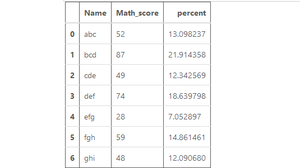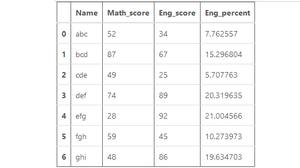Related Articles
How to calculate the Percentage of a column in Pandas ?
• Last Updated : 28 Jul, 2020

A Percentage is calculated by the mathematical formula of dividing the value by the sum of all the values and then multiplying the sum by 100. This is also applicable in Pandas Dataframes. Here, the pre-defined sum() method of pandas series is used to compute the sum of all the values of a column.

Syntax: Series.sum()

Return: Returns the sum of the values.

Formula:

```df[percent] = (df['column_name'] / df['column_name'].sum()) * 100
```

Example 1:

## Python3

 `# Import required libraries ` `import` `pandas as pd ` `import` `numpy as np ` `  `  `# Dictionary ` `df1 ``=` `{ ` `     ``'Name'``: [``'abc'``, ``'bcd'``, ``'cde'``, ` `             ``'def'``, ``'efg'``, ``'fgh'``, ` `             ``'ghi'``], ` `     ``'Math_score'``: [``52``, ``87``, ``49``, ` `                   ``74``, ``28``, ``59``, ` `                   ``48``]} ` `  `  `# Create a DataFrame ` `df1 ``=` `pd.DataFrame(df1,  ` `                   ``columns ``=` `[``'Name'``, ` `                             ``'Math_score'``]) ` ` `  `# Calculating Percentage ` `df1[``'percent'``] ``=` `(df1[``'Math_score'``] ``/`  `                  ``df1[``'Math_score'``].``sum``()) ``*` `100` ` `  `# Show the dataframe ` `df1 `

Output:Example 2:

## Python3

 `# Import pandas library ` `import` `pandas as pd ` `  `  `# Dictionary ` `df1 ``=` `{ ` `     ``'Name'``: [``'abc'``, ``'bcd'``, ``'cde'``, ` `             ``'def'``, ``'efg'``, ``'fgh'``, ` `             ``'ghi'``], ` `      ``'Math_score'``: [``52``, ``87``, ``49``, ` `                    ``74``, ``28``, ``59``, ` `                    ``48``], ` `       ``'Eng_score'``: [``34``, ``67``, ``25``,  ` `                    ``89``, ``92``, ``45``, ` `                    ``86``] ` `} ` `  `  `# Create a DataFrame ` `df1 ``=` `pd.DataFrame(df1,  ` `                   ``columns ``=` `[``'Name'``, ` `                   ``'Math_score'``,``'Eng_score'``]) ` ` `  `# Calculate Percentage ` `df1[``'Eng_percent'``] ``=` `(df1[``'Eng_score'``] ``/`  `                      ``df1[``'Eng_score'``].``sum``()) ``*` `100` `# Show the dataframe ` `df1 `

Output:Attention geek! Strengthen your foundations with the Python Programming Foundation Course and learn the basics.

To begin with, your interview preparations Enhance your Data Structures concepts with the Python DS Course.

My Personal Notes arrow_drop_up
Recommended Articles
Page :